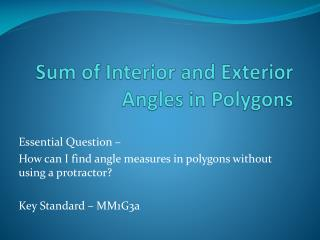DownloadDownload PresentationSum of Interior and Exterior Angles in Polygons

# Sum of Interior and Exterior Angles in Polygons

Download Presentation## Sum of Interior and Exterior Angles in Polygons

- - - - - - - - - - - - - - - - - - - - - - - - - - - E N D - - - - - - - - - - - - - - - - - - - - - - - - - - -
##### Presentation Transcript

1. Sum of Interior and Exterior Angles in Polygons Essential Question – How can I find angle measures in polygons without using a protractor? Key Standard – MM1G3a

2. Polygons • A polygon is a closed figure formed by a finite number of segments such that: 1. the sides that have a common endpoint are noncollinear, and 2. each side intersects exactly two other sides, but only at their endpoints.

3. Nonexamples

4. Polygons • Can be concave or convex. Concave Convex

5. Polygons are named by number of sides Triangle 3 4 Quadrilateral Pentagon 5 Hexagon 6 Heptagon 7 8 Octagon 9 Nonagon 10 Decagon 12 Dodecagon n n-gon

6. Regular Polygon • A convex polygon in which all the sides are congruent and all the angles are congruent is called a regular polygon.

7. Draw a:  Quadrilateral  Pentagon  Hexagon  Heptagon  Octogon • Then draw diagonals to create triangles. • A diagonal is a segment connecting two nonadjacent vertices (don’t let segments cross) • Add up the angles in all of the triangles in the figure to determine the sum of the angles in the polygon. • Complete this table

8. 3 1 180° 4 2 2 · 180 = 360° 5 3 3 · 180 = 540° 4 4 · 180 = 720° 6 7 5 5 · 180 = 900° 8 6 6 · 180 = 1080° n n - 2 (n – 2) · 180°

9. Polygon Interior Angles Theorem The sum of the measures of the interior angles of a convex n-gon is (n – 2) • 180. Examples – • Find the sum of the measures of the interior angles of a 16–gon. • If the sum of the measures of the interior angles of a convex polygon is 3600°, how many sides does the polygon have. • Solve for x. (16 – 2)*180 = 2520° (n – 2)*180 = 3600 180n = 3960 180 180 n = 22 sides 180n – 360 = 3600 + 360 + 360 (4 – 2)*180 = 360 4x - 2 108 108 + 82 + 4x – 2 + 2x + 10 = 360 6x = 162 6 6 2x + 10 82 6x + 198 = 360 x = 27

10. There are two sets of angles formed when the sides of a polygon are extended. • The original angles are called interior angles. • The angles that are adjacent to the • interior angles are called exterior angles. • These exterior angles can be formed when any side is extended. • What do you notice about the interior angle and the exterior angle? • What is the measure of a line? • What is the sum of an interior angle with the exterior angle? Draw a quadrilateral and extend the sides. They form a line. 180° 180°

11. If you started at Point A, and followed along the sides of the quadrilateral making the exterior turns that are marked, what would happen? You end up back where you started or you would make a circle. What is the measure of the degrees in a circle? A D B C 360°

12. Polygon Exterior Angles Theorem • The sum of the measures of the exterior angles of a convex polygon, one at each vertex, is 360°. • Each exterior angle of a regular polygon is 360 n where n is the number of sides in the polygon

13. Example Find the value for x. Sum of exterior angles is 360° (4x – 12) + 60+ (3x + 13) + 65 + 54+ 68 = 360 7x + 248 = 360 – 248 – 248 7x = 112 7 7 x = 12 (4x – 12)⁰ 68⁰ 60⁰ 54⁰ (3x + 13)⁰ 65⁰ What is the sum of the exterior angles in an octagon? What is the measure of each exterior angle in a regular octagon? 360° 360°/8 = 45°

14. Classwork/Homework Textbook: Read and study p298-299 Complete p300-301 (1-21) Show your work!